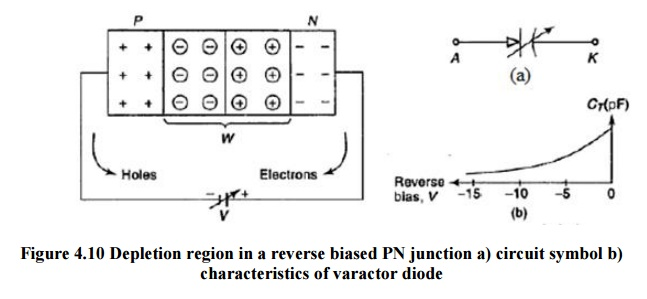Home | | Electronic Devices | Varactor Diode

# Varactor Diode

Varactors are operated in a reverse-biased state. No current flows, but since the thickness of the depletion zone varies with the applied bias voltage, the capacitance of the diode can be made to vary.

VARACTOR DIODE

Varactors are operated in a reverse-biased state. No current flows, but since the thickness of the depletion zone varies with the applied bias voltage, the capacitance of the diode can be made to vary. Generally, the depletion region thickness is proportional to the square root of the applied voltage; capacitance is inversely proportional to the depletion region thickness. Thus, the capacitance is inversely proportional to the square root of applied voltage.

All diodes exhibit this phenomenon to some degree, but varactor diodes are manufactured specifically to exploit this effect and increase the capacitance (and thus the range of variability), whereas most ordinary diode fabrication strives to minimize the capacitance.

A varactor diode is best explained as a variable capacitor. Think of the depletion region as a variable dielectric. The diode is placed in reverse bias. Junction capacitance is present in all reverse biased diodes because of the depletion region.Figure 4.10 Depletion region in a reverse biased PN junction a) circuit symbol b) characteristics of varactor diode

They are also called voltage-variable capacitance diodes. A Junction diode which acts as a variable capacitor under changing reverse bias is known as VARACTOR DIODE.A varactor diode is specially constructed to have high resistance under reverse bias. Capacitance for varactor dide are Pico farad. (10-12 ) range.Curve between Reverse bias voltage Vr across varactor diode and total junction capacitance CT and CT can be changed by changing Vr.

Applications

·   Junction  capacitance  is optimized in  a varactor         diode  and  is  used  fo r  high  frequencies  and switching applications.

·        Varactor diodes are often used for electronic tuning applications in FM radio s and televisions.

·        Varactors are used as voltage-controlled capacitors. They are commmonly used in voltage-controlled oscillators, parametric amplifiers, and frequency multipliers.

Study Material, Lecturing Notes, Assignment, Reference, Wiki description explanation, brief detail
Electronic Devices : Special Semiconductor Devices : Varactor Diode |